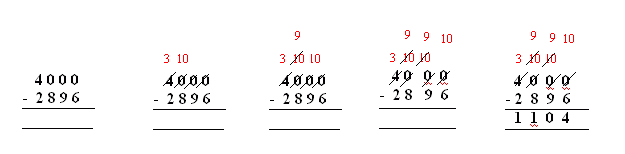Name: ___________________Date:___________________

 Email us to get an instant 20% discount on highly effective K-12 Math & English kwizNET Programs!

### Grade 4 - Mathematics Subtraction Across Zeroes

 Sometimes when you need to regroup, there is a zero in the next place. Then you need to regroup or rearrange the numbers in a different way. Method: Line up the numbers in their place value columns. Subtract the digits in the ones place. Since you cannot take away from zero, you need to regroup. That is borrow from tens place. If the tens digit is also zero then borrow from hundreds digit. If the hundreds digit is also zero then borrow from thousands digit and so on... After regrouping or rearranging subtract each column starting with the ones, tens, hundreds and so on.... Example:Regroup the thousands digit: 4 thousands as 3 thousands and 10 hundreds. Regroup the hundreds digit: 10 hundreds as 9 hundreds and 10 tens. Regroup the tens digit: 10 tens as 9 tens and 10 ones. Subtracting each column starting with ones, tens, hundreds and thousands gives 1104 Answer: 1104 Directions: Subtract the following. Show your work with regrouping numbers. Also write at least 5 examples of your own. For more worksheets: 1) Click Here 2) Refresh every time and you will see different worksheet for unlimited practise.Name: ___________________Date:___________________

### Grade 4 - Mathematics Subtraction Across Zeroes

 Question 1: 9000 - 6215

 Question 2: 9000 - 7483

 Question 3: 9000 - 7627

 Question 4: 9000 - 2921

 Question 5: 9000 - 8859

 Question 6: 9000 - 8359

 Question 7: 9000 - 8246

 Question 8: 9000 - 6920

 Question 9: 9000 - 3529

 Question 10: 9000 - 1732

 Question 11: 9000 - 3427

 Question 12: 9000 - 2564

 Question 13: 9000 - 4584

 Question 14: 9000 - 5729

 Question 15: 9000 - 2282

 Question 16: 9000 - 6188

 Question 17: 9000 - 1703

 Question 18: 9000 - 4590

 Question 19: 9000 - 6193

 Question 20: 9000 - 8724

 Question 21: 9000 - 5430

 Question 22: 9000 - 1043

 Question 23: 9000 - 5282

Question 24: This question is available to subscribers only!

Question 25: This question is available to subscribers only!

Question 26: This question is available to subscribers only!

Question 27: This question is available to subscribers only!

Question 28: This question is available to subscribers only!